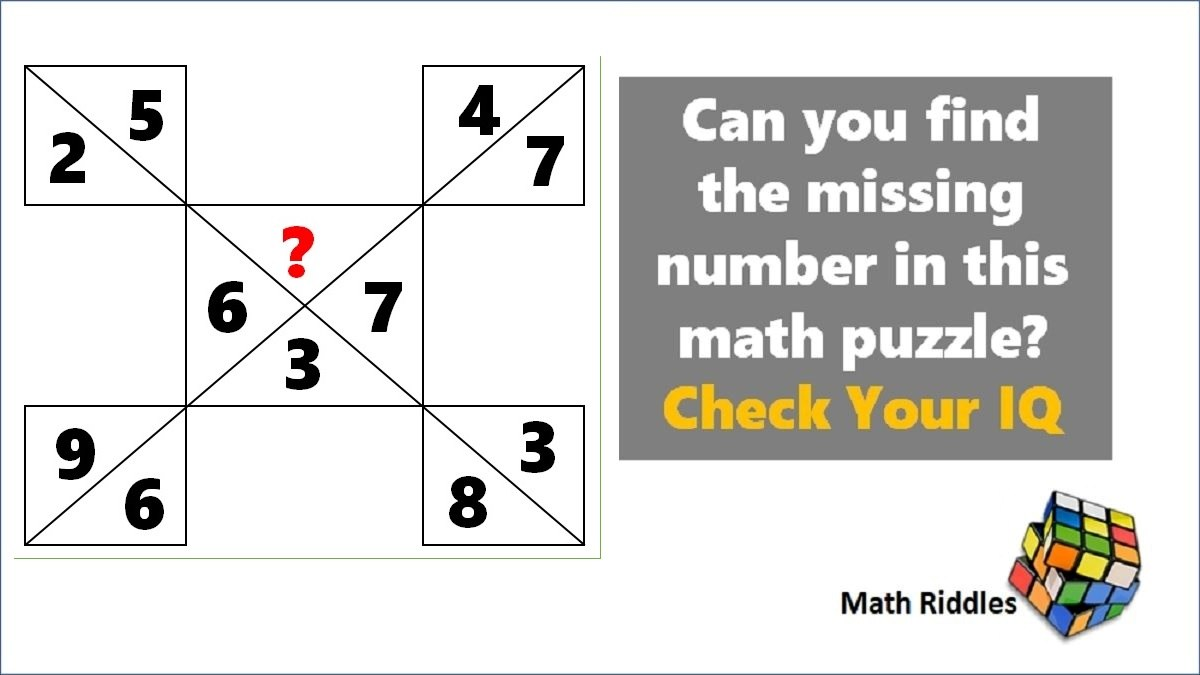# Math Riddles: Test Your IQ, Find The Missing Numbers Math Puzzles

Math Riddles Challenge: Can you find the missing number in this math puzzle? Check Your IQMath Riddles: Test Your IQ, Find The Missing Numbers Math Puzzles

Math Riddles are really fun way to test your maths skills. We another set of tricky math puzzle where one has to find the missing number in the place of the question mark. Today, we have two interesting math puzzles. Let us see if you can find the missing number in 30 seconds each.

## Solution

Logic Puzzle #1

Solution

We will solve this math puzzle in two parts. Let us begin with first part (LHS).

=> 2 + 6 + 9 = 17

=> 6 + 3 + 8 = 17

Let us similarly solve the second part (RHS).

=> 7 + 7 + 3 = 17

So far, we understood that the sum of all equations on both LHS and RHS should be 17.

Hence,

=> 5 + ? + 4 = 17

=> x + 9 = 17

=> x = 17 – 9 = 8

Logic Puzzle #2

Solution

First, let us understand the pattern in which other two triangles have been solved.

Similarly, we shall solve the triangle to find the missing number in place of the question mark.

## Tell us in comments: Did you solve these find missing number puzzles correctly in 30 seconds each?

Check out more math puzzles!

Also Read: Math Riddles: Missing Number Math Puzzle, IQ and Aptitude Test

Also Read: Math Riddles: Can You Find the Logic in this Magic Square 3×3?

Also Read: Math Riddles: Find the Value of X in this Algebra Equation

Get the latest General Knowledge and Current Affairs from all over India and world for all competitive exams.
खेलें हर किस्म के रोमांच से भरपूर गेम्स सिर्फ़ जागरण प्ले पर